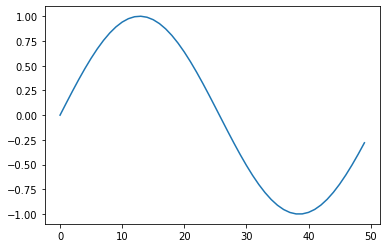This page was generated from docs/source/code_cells.ipynb, with configuration: sphinx_ipypublish_all.ext

Toggle Input Cells

Toggle Output Cells

# 2. Writing Code and Formatting Output¶

IPyPublish utilises metadata to mark-up the notebook with information on how output should be represented in the converted notebook, as shown in Fig. 2.1.

:

%matplotlib inline
import matplotlib.pyplot as plt
import numpy as np
plt.plot(np.sin(np.linspace(0, 6)))
plt.show()

Matplotlib is building the font cache; this may take a moment.Fig. 2.1 This is a Matplotlib figure, with a caption, a label and a set width

The PDF representation of this notebook

## 2.1. Converting Notebooks to Pure Python¶

To write code, we can work in the conventional Jupyter Notebook environment, or we can use jupytext, to convert between a notebook and the pure python percent format

$jupytext --to py:percent notebook.ipynb$ jupytext --to notebook notebook.py            # overwrite notebook.ipynb

## 2.7. Equations (with ipython or sympy)¶

An ipython and sympy equation Eq. 2.7.1 and Eq. 2.7.2.

:

from IPython.display import Latex
Latex('$$a = b+c$$')

:

\begin{equation} a = b+c \end{equation}
:

Code Block 2.7.1 The plotting code for a sympy equation Eq. 2.7.2.
sym = nb_setup.setup_sympy()
f = sym.Function('f')
y = sym.Function('y')
n = sym.symbols(r'\alpha')
f = y(n)-2*y(n-1/sym.pi)-5*y(n-2)
sym.rsolve(f,y(n),[1,4])

:

\begin{equation} \left(\sqrt{5} i\right)^{\alpha} \left(\frac{1}{2} - \frac{2 \sqrt{5} i}{5}\right) + \left(- \sqrt{5} i\right)^{\alpha} \left(\frac{1}{2} + \frac{2 \sqrt{5} i}{5}\right) \end{equation}

## 2.8. IPywidgets¶

ipywidgets can be added to the notebook, to create interactive elements. These widgets are preserved in sphinx HTML outputs.

:

import ipywidgets
slider1 = ipywidgets.FloatSlider()
slider1.description = "Slide Me"
slider1


Multiple views of the same widget can be created:

:

slider1


Using jslink, widgets can also be synced, without the need for an active python kernel:

:

slider2 = ipywidgets.BoundedFloatText()
(slider1, 'value'),
(slider2, 'value'))
slider2


For more complex examples see: jupyter.org/widgets

## 2.9. Object Output Formats¶

The format of the Jupyter Notebook file allows for the storage of a single output in multiple formats. This is taken advantage of by packages such as matplotlib and pandas, etc to store a figure/table in both latex and html formats, which can then be selected by ipypublish based on the document type required.

Sometimes a user may wish to have greater control over the output format and/or which output types are to be stored. It it possible to achieve this via the Jupyter display function. For example, if we wanted to display a pandas.DataFrame table without the index column, such that it can be output to both a pdf and html document:

:

from IPython.display import display
df = pd.DataFrame(np.random.random((3, 3)))
latex = df.to_latex(index=False)
html = df.to_html(index=False)
display({'text/latex': latex,
'text/html': html}, raw=True)


0

1

2

0.807925

0.733988

0.803516

0.636501

0.885510

0.340308

0.259823

0.665477

0.687906

If you wish to create your own object with multiple output formats, you should create a class with multiple _repr_*_() methods:

:

class MyObject(object):
def __init__(self, text):
self.text = text

def _repr_latex_(self):
return "\\textbf{LaTex: " + self.text + "}"

def _repr_html_(self):
return "<b>HTML: " + self.text + "</b>"

MyObject('hallo')

:

HTML: hallo

## 2.10. Multiple Outputs from a Single Code Cell¶

Similarly, with the Jupyter display functionality, you can control the output metadata for multiple outputs in a single code cell:

:

from IPython.display import display
from IPython.display import display_latex
from IPython.display import display_markdown

x = np.linspace(0, 3.42)

for i in range(1,3):

display_markdown(
'### Code Created Heading {0}'.format(i), raw=True)

fig, ax = plt.subplots()
ax.plot(x, np.sin(x*i))
'figure': {
plt.close()



### 2. Code Created Heading 1¶Fig. 2.10.1 Code Created Heading 1

### 2. Code Created Heading 2¶Fig. 2.10.2 Code Created Heading 2